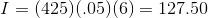# PSAT Math : How to find simple interest

## Example Questions

### Example Question #1 : Interest

Dan took out a $1200 loan at a rate of 3% simple interest a year. What is the amount of interest accrued for one month? Possible Answers: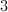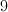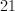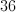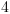Correct answer:Explanation:$1200 is the amount that he took out.

3% annually would yield an interest amount of $36. Therefore, each month, he would be paying$3 a month.

### Example Question #2 : Interest

Peter wanted to buy a car and borrowed $2,500 from the bank at 5% simple interest per year. He was able to pay back the loan in one lump sum at six months. What was the total amount he paid the bank? Possible Answers: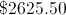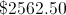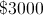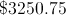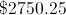Correct answer:Explanation: Simple interest is given by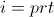where= interest,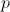= principal,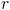= rate, and= time in years.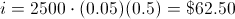Remember, six months is the same as half of a year. The total paid back to the bank is the principle plus the interest, or$2,562.50.

I have $100 in my bank. After one year, I have withdrawn a total of$20 and I still have $85 in the bank. What is the yearly interest rate of my bank account? Possible Answers: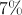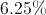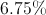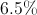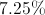Correct answer:Explanation: Since I have withdrawn$20, that means I only have $80 in my account.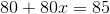imples that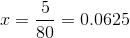which is 6.25%. ### Example Question #4 : Interest You really want to buy a car that costs$15,000 but you only have $5,000 saved. Rather than getting a bank loan, your parents offer to lend you the extra money but will require you to pay them back with 4.3% interest. How much will car cost in total after you have paid your parents back? Possible Answers: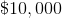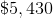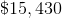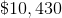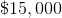Correct answer:Explanation: Amount borrowed =$15,000  $5,000 =$10,000

Interest of 4.3% = $10,000 * 0.043 =$430

Total cost of car = $5000 +$10000 + $430 =$15,430

### Example Question #5 : Interest

Ted works over the summer and makes $9 per hour. He works for 20 hours each week for 10 weeks. After paying 10% in taxes, he buys a bike for$500 and puts the rest of his money in the bank.

If Ted's bank pays 5% interest on the total sum once per year, and Ted doesn't add or remove anything from the account, how much money (rounded to the nearest cent) will Ted have in 5 years?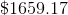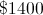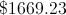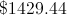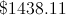Explanation:

To start, we see that Ted works for 20 hours per week for 10 weeks.  This means he works a total of 200 hours over the summer.  He is paid $9 dollars each hour. Therefore he makes$9 x 200 hours = $1800 total over the summer. He then has to pay 10% in taxes, or .1 x$1800, leaving him with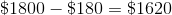.  He buys the bike for $500, so he has$1620 - $500 =$1,120 to deposit at the bank.

Now we have to find out how much he will have 5 years later.  Since the bank pays 5% interest each year, we know that he will make 5% of what he has each year.  He starts with $1,120. 5% of this value is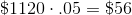. Adding this to the original$1,120 gives us \$1,176.

This is also the same as multiplying instead by 1.05.  We repeat this step 5 times, giving us: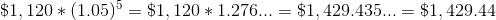### Example Question #1 : How To Find Simple Interest

Jane borrows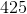dollars from the bank.  Her loan has an annual interest rate ofpercent. How much interest will she owe afteryears? (Use a simple interest calculation)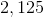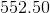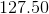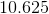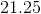Explanation:

Use the simple interest formula:

Interest equals the initial amount multiplied by the annual interest rate multiplied by the number of years: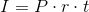Let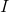= interest owed

Let= principal (aka the initial amount of the loan)

Let= interest rate

Let= years passed

Therefore: